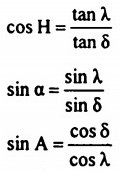top of pageSearch

# Survey (321-340)

Updated: Apr 25, 2020

321 Geographical map 1 cm = 160 km Topographical map 1 cm = 2.5 km Location map 1 cm = 5m to 25 m Forest map 1 cm = 0.25 km

322 Which one of the following is a conventional sign for North Line in surveying323.A Dumpy level is set up with its eyepiece vertically over a peg A. The height from the top of peg A to the centre of the eyepiece is 1.540 m and the reading on

peg B is 0.705 m. The level is then set up over peg B. The height of the eyepiece

above peg B is 1.490 m and the reading on A is 2.195 m. the difference in level

between A and B is 0.770 m

324.If the cross-sectional areas of an embankment at 30 m intervals are 20, 40,

60, 50 and 30 m² respectively, then the volume of the embankment on the basis of prismoidal rule, is

5300 m³

325.If the bearing of a line AB is N 60° 30' and that of BC is 122° of a closed traverse

ABCDE, then the measure of the interior angle B is 118° 30'

326.The techeometer focal length of object glass is 20 cm, the distance between the object glass and trunnion axis is 10 cm and the spacing between the outer lines of diaphragm axis is 4 mm. If the staff intercepts are 1.000 (top) and 2.500

(middle) when the line of collimation is perfectly horizontal, then the horizontal

distance between the staff station and instrument station will be 150.3

327. If the coordinates of A are 100 N and 200 E and those of C are 100 S and 200 E,

then the length AC is 200.00

328. Which one of the following instruments is used in plane table surveying for the measurement of horizontal and vertical distances directly ? Plain alidade

329. The deflection angle between the tangents drawn at the ends of a transition curve is 7°. The radius of the curve at the end is 400 m. What is the length of the transition curve ? 97.74 m

330. A transition curve is required for a circular curve of 200 m radius, the gauge being 1.5 m and maximum superelevation restricted to 15 cm. The transition is to be designed for a velocity such that no lateral pressure is imposed on the rails and the rate of gain of radial acceleration is 30 cm/s². The required length of transition curve will work out to be 46 m

331. Which of the following elements of a simple curve are correctly matched?

(R is the radius and Δ is the deflection angle)

1. Tangent length R tan Δ/2

2. Mid-ordinate ........ R ver sin Δ/2

332. A parabolic vertical curve is set out connecting a + 0.7% grade to a - 0.6%

grade. The chainage and R.L. of the point of intersection are 1000 m and 250 m

respectively. The admissible rate of change of grade is 0.05% per 20 m. The

chainage of the tangent points will be 740 and 1260

333. A, B and C are triangulation stations and S is the satellite station for B. The true angle at B is given by α = θ - β1 + β2 where β1 = SAB, β2 = SCB and θ = ASC. The

position of S is

between AC and B

334. Consider the following statements associated with triangulation survey

1. In tertiary triangulation, the length of the sides is in the range of 10 to 25 km.

2. The length of the base line in primary triangulation is 8 to 12 km.

335. Which one of the following is the combined .angular correction for angles of

elevation in trigonometrical levelling ? (Symbols have the usual meanings)336 zero and tends to change from - ve to + ve - At the first point of Libra Zero and tends to change from + ve to - ve - At the first point of Aries +23° 27 - At Summer Solstice -23° 27 - At Winter Solstice

337. A star culminates in zenith when δ = θ

338. If α, H, A and δ are the altitude, hour angle, azimuth and declination of a circumpolar star at its elongation in latitude λ, then which one of the following relations would hold good?339. When the latitude is 56°15' N aftd declination is 17°30' S, the 'zenith

distance* at the upper culmination of the star will be 73° 45'

340.If the parallax difference between the top and bottom of a tree is measured as 1.32 mm on a stereo-pair of photos taken at 3000 m above ground and the average photobase is 66 mm, then the height of the tree will be 60.00 m